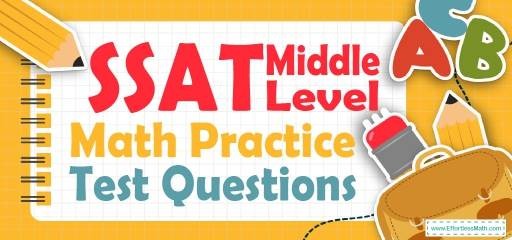# SSAT Middle Level Math Practice Test QuestionsPreparing your student for the SSAT Middle-Level Math test? Try these free SSAT Middle-Level Math Practice questions. Reviewing practice questions is the best way to brush up on your student’s Math skills. Here, we walk you through solving 10 common SSAT Middle-Level Math practice problems covering the most important math concepts on the SSAT Middle-Level Math test.

These SSAT Middle-Level Math practice questions are designed to be similar to those found on the real SSAT Middle-Level Math test. They will assess your student’s level of preparation and will give you a better idea of what your student needs to study on his/her exam.

## 10 Sample SSAT Middle Level Math Practice Questions

1- In a group of $$5$$ books, the average number of pages is $$24$$. Mary adds a book with $$36$$ pages to the group. What is the new average number of pages per book?

A. 20

B. 22

C. 24

D. 26

E. 30

2- A football team won exactly $$70\%$$ of the games it played during last session. Which of the following could be the total number of games the team played last season?

A. 49

B. 40

C. 32

D. 12

E. 9

3- If a gas tank can hold $$35$$ gallons, how many gallons does it contain when it is $$\frac{2}{5}$$ full?

A. 50

B. 125

C. 62.5

D. 14

E. 8

4- What is the value of $$?$$ in the following figure? (Figure is not drawn to scale)

A. 150

B. 145

C. 125

D. 105

E. 85

5- The capacity of a red box is $$20\%$$bigger than the capacity of a blue box. If $$36$$ books can be put in the red box, how many books can be put in the blue box?

A. 15

B. 20

C. 24

D. 30

E. 32

6- A taxi driver earns $$8$$ per 1-hour work. If he works $$10$$ hours a day and in $$1$$ hour he uses $$2$$-liters petrol with price $$1$$ for $$1$$-liter. How much money does he earn in one day?

A. $90 B.$88

C. $70 D.$60

E. $56 7- Which of the following is less than $$\frac{1}{5}$$? A. $$\frac{1}{4}$$ B. 0.5 C. $$\frac{1}{7}$$ D. 0.28 E. 0.31 8- Amy and John work in the same company. Last month, both of them received a raise of $$20$$ percent. If Amy earns $$30.00$$ per hour now and John earns $$28.80$$, Amy earned how much more per hour than John before their raises? A.$8.25

B. $4.25 C.$3.00

D. $2.25 E.$1.00

9- Three people can paint $$3$$ houses in $$12$$ days. How many people are needed to paint $$6$$ houses in $$6$$ days?

A. 6

B. 8

C. 12

D. 16

E. 20

10- If $$?×6−3=12$$ then $$?=?$$

A. 4

B. 12

C. 13

D. 14

E. 18

## Best SSAT Middle LevelMath Prep Resource for 2022

1- D
In a group of $$5$$ books, the average number of pages is $$24$$. Therefore, the sum of pages in all $$5$$ books is $$(5×24=120)$$. Mary adds a book with $$36$$ pages to the group. Then, the sum of pages in all 6 books is $$(5×24+36=156)$$. The new average number of pages per book is:$$\frac{156}{6}=26$$

2- B
Choices A, C, D, and E are incorrect because $$70\%$$ of each of the numbers is a non-whole number.
A. $$49, 70\%$$ of $$49 = 0.70×49=34.3$$
B. $$40, 70\%$$ of $$40=0.70×40=28$$
C. $$32, 70\%$$ of $$32=0.80×32=22.4$$
D. $$12, 70\%$$ of $$12=0.70×12=8.$$
E. $$9, 70\%$$ of $$9=0.80×9=6.3$$

3- D
$$\frac{2}{5}×35=\frac{70}{5}=14$$

4- A
$$x=25+125=150$$

5- D
The red box is $$20\%$$ bigger than the blue box. Let $$x$$ be the capacity of the blue box. Then:
$$x+20\%$$ of $$x=36→1.2x=36→x=\frac{36}{1.2}=30$$

6- D
$$8×10=80$$, Petrol use: $$10×2=20$$ liters, Petrol cost: $$20×1=20$$
Money earned: $$80-20=60$$

7- C
From the choices provided, only C $$(\frac{1}{7})$$ is less than $$\frac{1}{5}$$.

8- E
Amy earns $$30.00$$ per hour now. $$30.00$$ per hour is $$20$$ percent more than her previous rate. Let $$x$$ be her rate before her raise. Then: $$x+0.20x=30→1.2x=30→x=\frac{30}{1.2}=25$$
John earns ($28.80) per hour now. $$28.80$$ per hour is $$20$$ percent more than his previous rate. Let $$x$$ be John’s rate before his raise. Then:$$x+0.20x=28.80→1.2x=28.80→x=\frac{28.80}{1.2}=24$$, Amy earned $$1.00$$ more per hour than John before their raises. 9- C Three people can paint $$3$$ houses in $$12$$ days. It means that for painting $$6$$ houses in $$12$$ days we need $$6$$ people. To paint $$6$$ houses in $$6$$ days, $$12$$ people are needed. 10- A $$N×(6-3)=12→N×3=12→N=4$$ Looking for the best resource to help you succeed on the SSAT Middle-Level Math test? ## The Best Books to Ace the SSAT Middle LevelMathTest ## Related to This Article ### More math articles ### What people say about "SSAT Middle Level Math Practice Test Questions - Effortless Math: We Help Students Learn to LOVE Mathematics"? No one replied yet. X 23% OFF Limited time only! Save Over 23% SAVE$5

It was $21.99 now it is$16.99